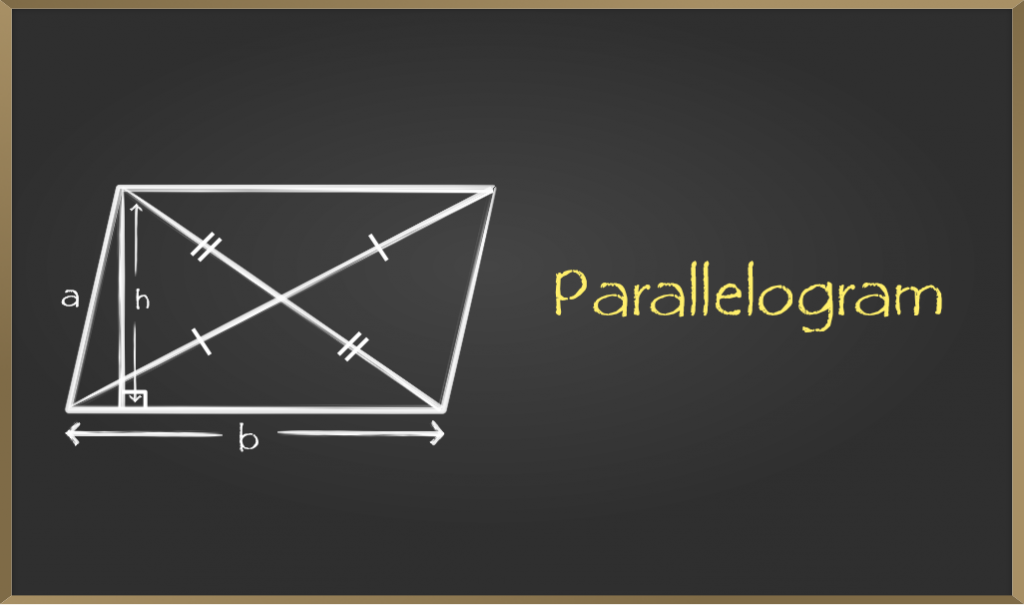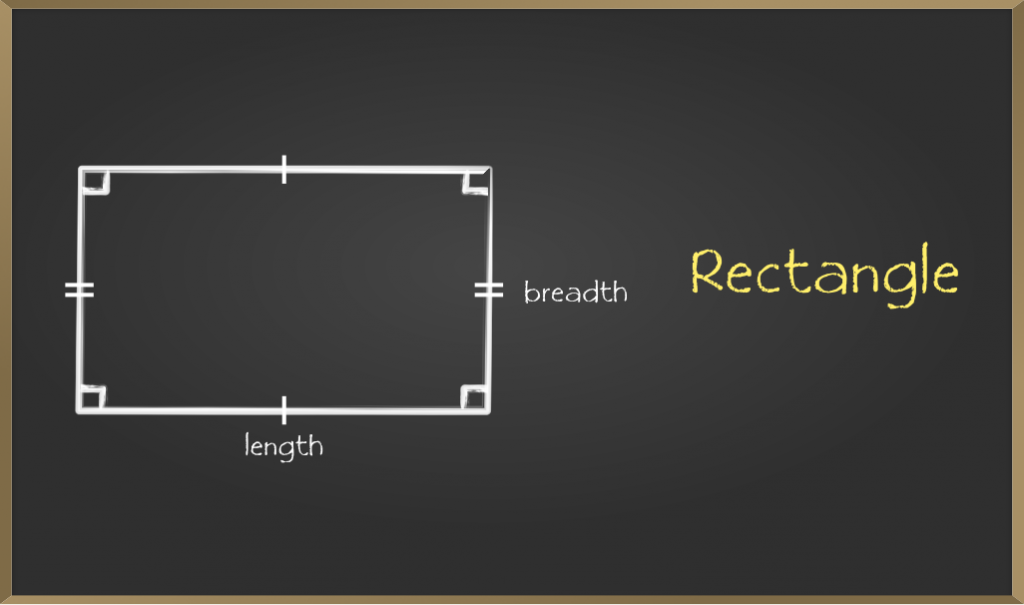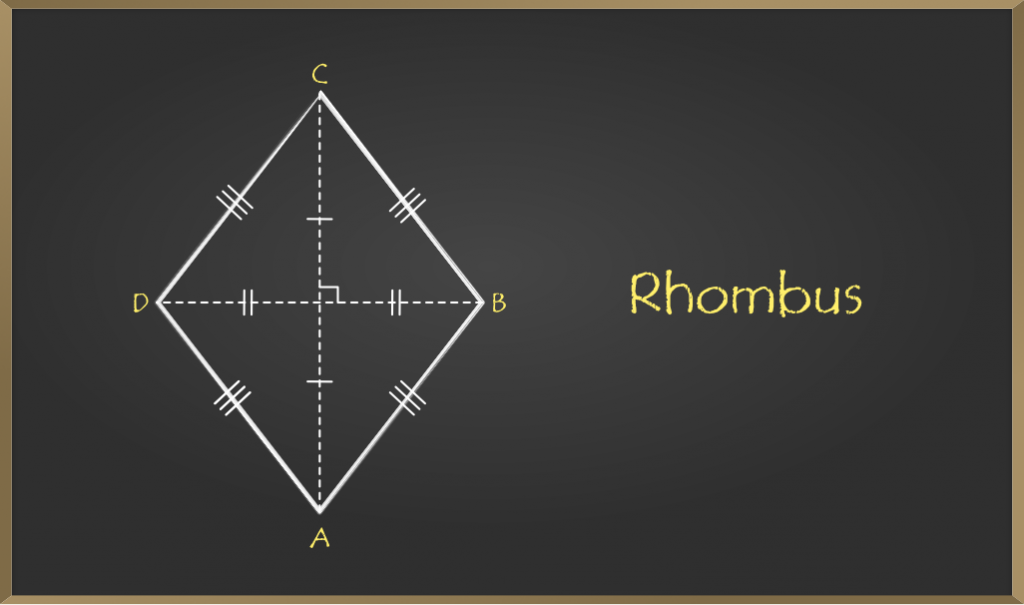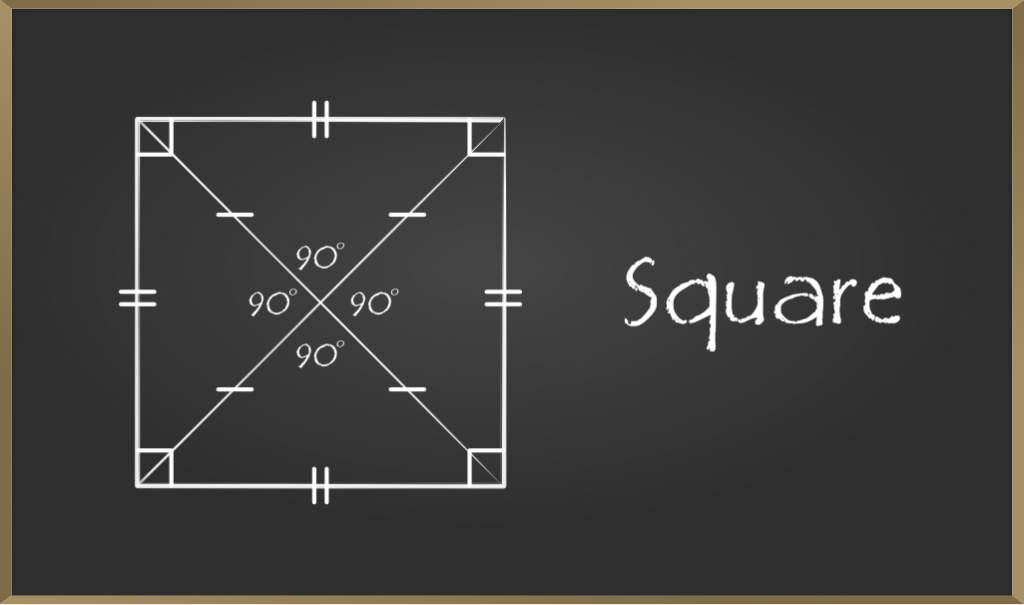Related Articles

# Types of Quadrilaterals – Some Special Parallelograms

• Last Updated : 21 Dec, 2020

Quadrilaterals can be defined as, types of polygons that have four sides, four vertices, and four angles along with a pair of diagonals. The Sum of interior angles of quadrilaterals is 360°. There are various kinds of quadrilaterals. As the name itself suggest the word is a combination of two Latin words ‘Quadri‘ means a variant of four, and ‘latus‘ means side. In this topic, we are going to study some special types of parallelogram like rectangle, square, rhombus.

A parallelogram is a special type of quadrilateral whose opposite side are equal and parallel.### Properties of Parallelogram

There are different properties of parallelograms.

1. Opposite sides are equal.
2. Opposite angle are equal
3. Diagonals bisect each other.
4. A diagonal of a parallelogram divides it into two congruent triangles.

### Some Special Parallelograms

Depending on the properties, there are three special types of parallelogram:

• Rectangle
• Rhombus
• Square

## Rectangle

A rectangle is a special type of parallelogram which has all the properties of parallelogram along with some different properties. Each angle of a rectangle must be a right angle, i.e. of 90°.### Properties of a Rectangle

Properties of a rectangle are similar to those of a parallelogram:

• Opposite Sides are parallel to each other.
• Opposite Sides of a rectangle are equal.
• Diagonals bisect each other
• Diagonals of the rectangle are equal.
• Each interior angle of a rectangle is equal i.e. 90°

Note: Rectangle has all the properties of parallelogram. So all rectangles are parallelogram but all parallelograms are not rectangle.

### Sample Problems on Rectangles

Problem 1: In rectangle ABCD, AO = 5cm. Find the length of diagonal BD. Also find the perimeter of rectangle if AB = 8cm and AD = 6cm.

Solution:

AO = OC = 5cm (diagonals bisect each other)

Therefore, AC = 10cm

BD = AC =10cm (diagonals of rectangle are equal)

Perimeter = AB + BC + CD + DA

=8 + 6 +8 +6 (opposite sides are equal)

= 28cm

Problem 2: In rectangle ABCD, ∠ABD = 3x – 7 and ∠CBA = 6x – 2. Find the value of x.

Solution:

Each angle of rectangle is 900

Therefore,

∠ABD + ∠CBA = 900

3x – 7 + 6x – 2 = 90

9x – 9 = 90

9x = 99

x = 11

Problem 3: In rectangle ABCD AO = 2x – 10 cm, OB = x + 4 cm. Find the length of diagonal BD

Solution:

In rectangle diagonals bisect each other and are equal.

Therefore, AO = OB

2x – 10 = x + 4

x = 14

OB = 14 + 4 = 18 cm

OD = 18 cm (as diagonals bisect each other)

Therefore, BD = 36 cm

## Rhombus

A quadrilateral that has all sides equal and opposite sides parallel is called Rhombus. Opposite angles of a rhombus are equal and diagonals of the Rhombus bisect each other perpendicularly. Note all rhombus are parallelograms but the reverse of this is not true.### Properties of a Rhombus

Properties of a rhombus are similar to those of a parallelogram:

• Opposite Sides are parallel to each other.
• All the sides of a rhombus are equal to each other.
• Diagonals bisect each other
• Opposite angles of a rhombus are equal.

Note: Rhombus is a parallelogram with all side equal

### Sample Problems on Rhombus

Problem 1: Diagonals of rhombus are 24cm and 10cm. Find the side of rhombus.

Solution:

AC = 24cm

BD = 10cm

Therefore, AO = 12cm and OB = 5cm (diagonals bisect each other)

In right-angled triangle AOB, (diagonals of rhombus are perpendicular)

AB2 = OA2 + OB2

AB2 = 122 + 52

AB2 = 144 + 25

AB2 = 169

AB = 13cm

Therefore, side of rhombus is 13cm.

Problem 2: In a rhombus one of the diagonals is equal to a side of the rhombus. Find the angles of rhombus.

Solution:

In rhombus PQRS PR = PQ (given)

Therefore, PQ = QR = RS = SP = PR (as all side of rhombus are equal)

In triangle PQR

PQ = QR = PR

Therefore, it is an equilateral triangle.

∠QPR = ∠Q = ∠QRP = 600

||ly ∠SPR = ∠S = ∠PRS = 600

Therefore, angles of rhombus are ∠P = 1200 , ∠Q = 600, ∠R = 1200, ∠S = 600

Problem 3: Derive the formula for are of rhombus.

Solution:

As diagonals of rhombus bisect each other at right angle.

In rhombus ABCD

area of triangle ABD = 1/2 * BD *AO (1/2 * base *height) ……….  (1)

area of triangle BCD = 1/2 * BD * CO ………………………………………….. (2)

Area of rhombus = Area of triangle ABD + area of triangle BCD

= 1/2 * BD * AO + 1/2 * BD * CO

= 1/2 * BD (AO + CO)

= 1/2 * BD * AC           (AE + CE = AC)

Therefore, area of rhombus = 1/2 * product of diagonals

## Square

A quadrilateral that has all sides equal and opposite sides parallel and all interior angles equal to 90° is called Square. Diagonals of a square bisect each other perpendicularly. Note that all squares are rhombus but not vice-versa### Properties of a Square

Properties of a square are similar to those of a rhombus:

• Opposite Sides are parallel to each other.
• All the sides of a square are equal to each other.
• Diagonals are perpendicular bisector of each other and are equal.
• All the angles of a square are equal and of 90° each.

### Sample Problems on Square

Problem 1: All rhombus are squares or all squares are rhombus. Which of these statements is correct and why?

Solution:

Square and rhombus both have all sides equal but a rhombus is called square if each of its angle is 900. So all squares can be called rhombus but converse is not true.

Problem 2: In the figure ROPE is a square. Show that diagonals are equal.

Solution

In Δ REP and Δ OEP

RE = OP (sides of square)

∠E = ∠P (each 900)

EP = EP (common)

Therefore, triangles are congruent by SAS criteria.

Therefore, RP = OE (c.p.c.t)

Therefore, diagonals of square are equal.

Attention reader! Don’t stop learning now. Join the First-Step-to-DSA Course for Class 9 to 12 students , specifically designed to introduce data structures and algorithms to the class 9 to 12 students

My Personal Notes arrow_drop_up